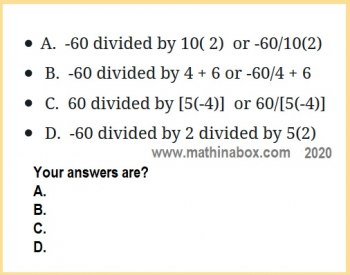# Why is multiplication completed first here: -12+8(2)/(4) but not here: -12+8/2(4)?

Posted on: April 30, 2020 Posted by: Susan Johnsey Comments: 1The order that we complete the 4 operations of mathematics has very specific rules. The rules are needed so that each math expression will have its own unique answer. When the rules are applied there is no confusion as to its value. Sometimes we can compute the right answer without knowing the rules! Look at these two. Everyone can get these right: 11(4) -10 or (6+8) – 13. Problems that require knowing the proper order are often seen on viral Facebook posts! Have you see some of them? But let us see how you do with these:

• A. -60 divided by 10( 2) or -60/10(2)
• B. -60 divided by 4 + 6 or -60/4 + 6
• C. 60 divided by [5(-4)] or 60/[5(-4)]
• D. -60 divided by 2 divided by 5(2)

Which of the above means – 3 and which means -12?  Which one has another number for its answer? Study the rules below after you find your answers for the above questions. We will see how you did.

The above problems require only knowing 3 of the 4 rules since I did not include any exponents. If we also have exponents then that will be the second rule. So what is the first rule? SEARCH for grouping symbols and complete the work inside them. If there are any nested ones (grouping symbols within other grouping symbols) then be sure to look within the inner ones first and work outward using all the rules. Grouping symbols are these pairs: ( ), and [ ], and { }, and | |, and also the long fraction bar and long radical symbol. Did I miss any?

Rules for Order are needed when using more than one of these: ADD, SUBTRACT, MULTIPLY, DIVIDE, and EXPONENTS. I hope you know this is just a list of the 4 operations and exponents. These are skills you need prior to using the ORDER of OPERATIONS. The list above is NOT the order to complete them. So WHAT is the ORDER?

1. SEARCH for grouping symbols that have work inside (start with the innermost). If long fraction bar found consider the top as a group and the bottom as another group. Work under the long radical symbols. You may need to follow all 4 rules on this step.
2. SEARCH for exponents (and radicals). [Warning for next 2 steps: No more searching. Each of these below are completed Left to Right.]
3. START on LEFT for divides and multiplies (go across only once completing each as you come to it). At this point, the only things left to do are adding and subtracting. Look at your expression. Is that correct?
4. START on LEFT for adds and subtracts (again, go across only once completing each as you come to it ) .

Now go back to the 4 problems above and redo them if you did not follow the rules. The answers: A. -12 B. -9 C. -3 D. -12

### Do you have questions? Here is a problem for you to try:

E. \qquad 335 – 6(4+2)^2 – 8^2

Where do we begin? See complete answer and work for problem E here. Use the back button to return here when you finish it.

W. 1

X. -4

Y. 11

Z. 19

## Let me know your questions.

### 1 people reacted on this

1.zoritoler imol says:

I have been surfing online greater than 3 hours lately, yet I by no means found any fascinating article like yours. It is lovely price enough for me. In my view, if all website owners and bloggers made good content as you probably did, the web will likely be much more useful than ever before.